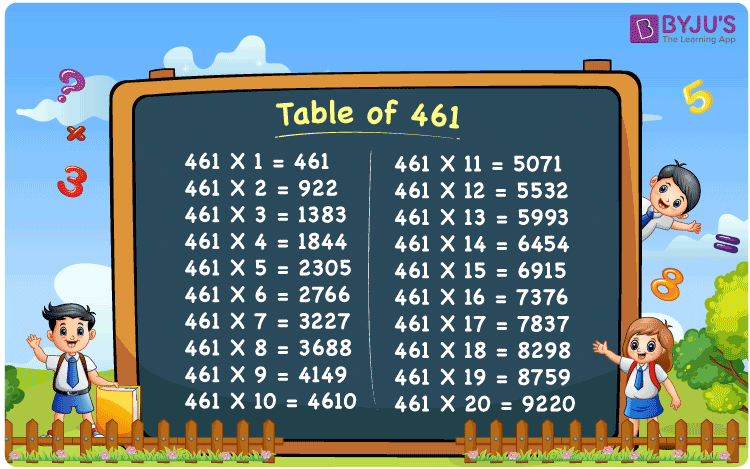Checkout JEE MAINS 2022 Question Paper Analysis : Checkout JEE MAINS 2022 Question Paper Analysis :

# Table of 461

The Table of 461 is the multiplication table which indicates the multiples of 461. The tables are used to calculate the numerical problems with ease. 461 times table can be generated by multiplying the number 461 by different whole numbers. We can also use the method of repeatedly adding 461 for n times to get the multiplication table of 461. Multiplication tables are very important to get a hold on the basic concepts discussed in Mathematics. Students can download the table of 461 from the below mentioned links.## What is the 461 Times Table?

461 times table can be formulated by repeated addition of 461 for n number of times. Students can learn how to design the table of 461 in both multiplication and addition form with the help of the below chart. Also, check the multiples of 461 for 10 times here.

 461×1 = 461 461 461×2 = 922 461 + 461 = 922 461×3 = 1383 461 + 461 + 461 = 1383 461×4 = 1844 461 + 461 + 461 + 461 = 1844 461×5 = 2305 461 + 461 + 461 + 461 + 461 = 2305 461×6 = 2766 461 + 461 + 461 + 461 + 461 + 461 = 2766 461×7 = 3227 461 + 461 + 461 + 461 + 461 + 461 + 461 = 3227 461×8 = 3688 461 + 461 + 461 + 461 + 461 + 461 + 461 + 461 = 3688 461×9 = 4149 461 + 461 + 461 + 461 + 461 + 461 + 461 + 461 + 461 = 4149 461×10 = 4610 461 + 461 + 461 + 461 + 461 + 461 + 461 + 461 + 461 + 461 = 4610

## Multiplication Table of 461

Students can make use of multiplication tables provided at BYJU’S to help themselves in solving the problems of textbooks in an easy and quick way. Learn the first 20 multiples of 461 from the below chart and enhance the skills, vital for examinations.

 461 × 1 = 461 461 × 2 = 922 461 × 3 = 1383 461 × 4 = 1844 461 × 5 = 2305 461 × 6 = 2766 461 × 7 = 3227 461 × 8 = 3688 461 × 9 = 4149 461 × 10 = 4610 461 × 11 = 5071 461 × 12 = 5532 461 × 13 = 5993 461 × 14 = 6454 461 × 15 = 6915 461 × 16 = 7376 461 × 17 = 7837 461 × 18 = 8298 461 × 19 = 8759 461 × 20 = 9220

## Solved Example on the Table of 461

Example 1:

The cost of a handbag is Rs 461. Calculate the cost of 9 such handbags.

Solution:

The cost of a handbag = Rs 461

Cost of 9 such handbags = 461 x 9 = 4149

Hence, the cost of 9 handbags is Rs 4149.

## Frequently Asked Questions on Table of 461

### Is 461 a perfect square?

No, 461 is not a perfect square.

### Mention the first five multiples of 461.

461, 922, 1383, 1844 and 2305 are the first five multiples of 461.

### What can be multiplied by 461 to get the value 3227?

As per the 461 times table, 461 times 7 is equal to 3227. Hence, 461 has to be multiplied by 7 times to get the value 3227.